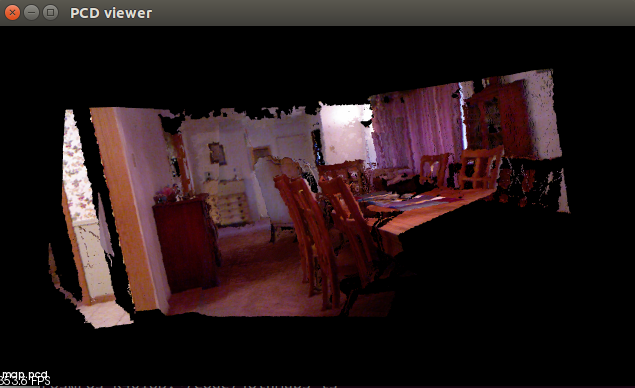# 深入學習 lsd-slam - 5

## 核心概念

$[x, y, z, q_x, q_y, q_z, q_w]$

## 實作

### 基本函式庫安裝

sudo add-apt-repository ppa:v-launchpad-jochen-sprickerhof-de/pcl
sudo apt-get update
sudo apt-get install libpcl-all


### 程式碼

#include <iostream>
#include <fstream>
using namespace std;

#include <opencv2/core/core.hpp>
#include <opencv2/highgui/highgui.hpp>
#include <Eigen/Geometry>
#include <boost/format.hpp>  // for formating strings
#include <pcl/point_types.h>
#include <pcl/io/pcd_io.h>
#include <pcl/visualization/pcl_visualizer.h>

int main( int argc, char** argv )
{
vector<cv::Mat> colorImgs, depthImgs;    // 彩色影像和深度影像
vector<Eigen::Isometry3d> poses;         // 相機 pose

ifstream fin("./pose.txt");
if (!fin)
{
cerr << "必須在有pose.txt的目錄下執行此程式" << endl;
return 1;
}

// 讀取彩色影像、深度影像以及對應的相機 pose
for ( int i=0; i<5; i++ )
{
boost::format fmt( "./%s/%d.%s" );

double data = {0};
for ( auto& d:data )
fin>>d;
Eigen::Quaterniond q( data, data, data, data );
Eigen::Isometry3d T(q);
T.pretranslate( Eigen::Vector3d( data, data, data ));
poses.push_back( T );
}

// 計算 point cloud 並接起來
// 指定相機內部參數
double cx = 325.5;
double cy = 253.5;
double fx = 518.0;
double fy = 519.0;
double depthScale = 1000.0;

cout << "正在將影像轉換為 point cloud ..." << endl;

// 定義 point cloud 使用的格式：這邊用的是XYZRGB
typedef pcl::PointXYZRGB PointT;
typedef pcl::PointCloud<PointT> PointCloud;

PointCloud::Ptr pointCloud( new PointCloud );
for ( int i=0; i<5; i++ )
{
cv::Mat color = colorImgs[i];
cv::Mat depth = depthImgs[i];
Eigen::Isometry3d T = poses[i];
for ( int v=0; v<color.rows; v++ )
for ( int u=0; u<color.cols; u++ )
{
//使用內部參數與深度值算出相機座標系下的 point cloud
unsigned int d = depth.ptr<unsigned short> ( v )[u]; // 深度值
if ( d==0 ) continue; // 深度為0表示沒有量到
Eigen::Vector3d point;
point = double(d)/depthScale;
point = (u-cx)*point/fx;
point = (v-cy)*point/fy;

//用外部參數轉換到世界座標系底下
Eigen::Vector3d pointWorld = T*point;
PointT p ;
p.x = pointWorld;
p.y = pointWorld;
p.z = pointWorld;
p.b = color.data[ v*color.step+u*color.channels() ];
p.g = color.data[ v*color.step+u*color.channels()+1 ];
p.r = color.data[ v*color.step+u*color.channels()+2 ];
pointCloud->points.push_back( p );
}
}

//儲存 point cloud
pointCloud->is_dense = false;
cout << "There are total " << pointCloud->size() << " points in the map.pcd." <<endl;
pcl::io::savePCDFileBinary("map.pcd", *pointCloud );
return 0;
}


### 編譯與執行

mkdir build
cd build
cmake ..
make
mv joinMap ../
cd ..


./joinMap
pcd_viewer map.pcd## 延伸閱讀

@pojenlai 演算法工程師，對機器人跟電腦視覺有少許研究，最近在學習看清事物的本質與改進自己的觀念